Home Practice
For learners and parents For teachers and schools
Textbooks
Full catalogue
Pricing SupportLog in

We think you are located in United States. Is this correct?

# Finding unknown angles on parallel lines

## Worked example 12.8: Working out unknown angles on parallel lines

Work out the sizes of the unknown angles, $$x$$, $$y$$ and $$z$$. Give reasons for your answers.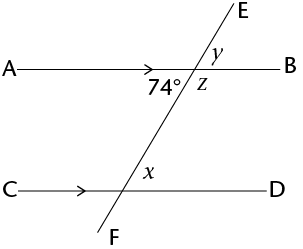Remember that you can use abbreviations when you give your reasons within your workings. For example, for ‘alternate angles of parallel lines AB and CD are equal’ you write (alt $$\angle$$s ; $$AB \parallel CD$$).

### Identify all the angles vertically opposite the given angle.

\begin{align} y &= 74^{\circ} &&(\text{vert opp }\angle) \end{align}

### Identify any angles that are alternate or corresponding to the given angle.

\begin{align} x &= 74^{\circ} &&(\text{alt}\angle\text{s; } AB \parallel CD) \end{align}

### Identify any angles that are adjacent to the given angle on a straight line.

\begin{align} z =180^{\circ}-74^{\circ}= 106^{\circ} &&(\angle\text{s on a straight line}) \\ z = 106^{\circ} \end{align}

Alternatively, we could have also solved the problem like this:

\begin{align} y &= 74^{\circ} && (\text{vert opp }\angle\text{s}) \\ x &= y &&(\text{corresp }\angle\; AB \parallel CD) \\ x &= 74^{\circ} \\ z +x &= 180^{\circ} &&(\text{co-int }\angle\; AB \parallel CD) \\ z +74^{\circ} &= 180 \\ z &= 180^{\circ}-74^{\circ} \\ z &= 106^{\circ} \end{align}
Exercise 12.6

Calculate the size of $$x$$ and $$y$$.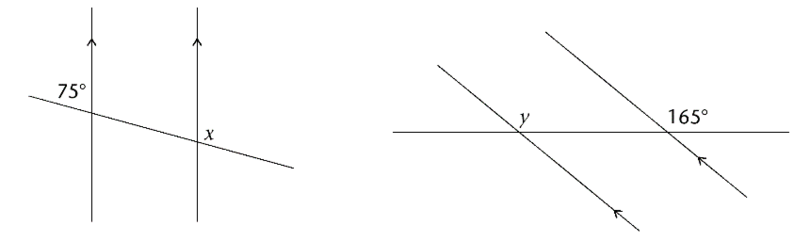\begin{align} \hat{x}_1& =75° &(\text{corresp }\angle\text{s})\\ 75°+x &=180° &(\angle\text{s on a straight line}) \\ x &= 180°-75° \\ x &= 105° \\ y &= 165°&(\text{corresp }\angle\text{s}) \end{align}

Calculate the size of $$p$$, $$q$$ and $$r$$.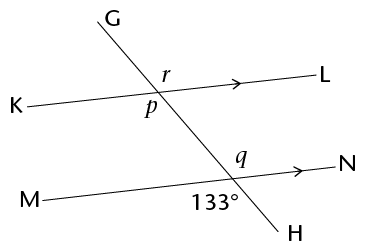\begin{align} p&= 133°&&(\text{corresp }\angle\text{s}; KL \parallel MN) \\ q&= 133°&& (\text{vert opp }\angle\text{s}) \\ r&= 133°&&(\text{corresp }\angle\text{s}; KL \parallel MN) \end{align}

Calculate the size of $$a$$, $$b$$, $$c$$ and $$d$$.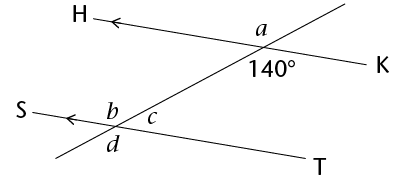\begin{align} a&= 140°&& (\text{vert opp }\angle\text{s}) \\ b&= 140°&&(\text{corresp }\angle\text{s}; HK \parallel ST) \\ d&= 140°&& (\text{vert opp }\angle\text{s}) \\ c&= 180°-140°&&(\angle\text{s on a straight line}) \\ c&= 40° \\ \end{align}

In the diagram, $$MP \parallel XY$$ and $$JK \parallel RS$$. Find the sizes of the marked angles.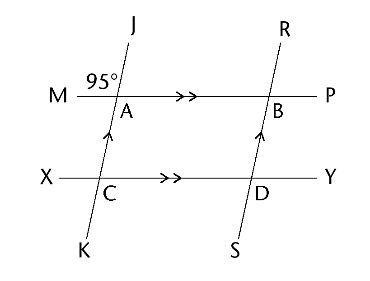\begin{align} A&= 95°&& (\text{vert opp }\angle\text{s}) \\ \\ B&= 95°&&(\text{corresp }\angle\text{s}; JK \parallel RS) \\ \\ C&= 95°&&(\text{corresp }\angle\text{s}; MP \parallel XY) \\ \\ D&= 95°&&(\text{corresp }\angle\text{s}; JK \parallel RS) \\ \\ \end{align}

Calculate the size of $$x$$, $$y$$ and $$z$$.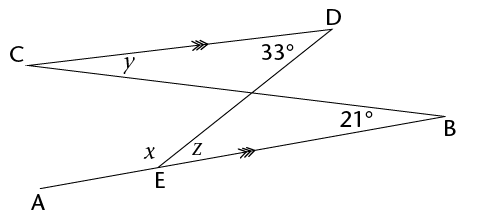\begin{align} z &=33° &&(\text{alt }\angle\text{s}; CD \parallel AB) \\ y &=21° &&(\text{alt }\angle\text{s}; CD \parallel AB) \\ x &= 180°-z&&(\angle\text{s on a straight line}) \\ x &= 180°-33° \\ x &= 147° \end{align}

Calculate the size of $$x$$.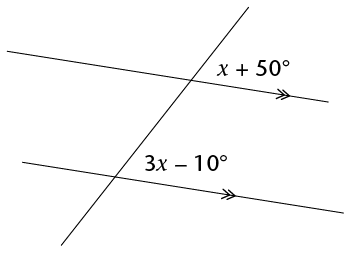\begin{align} x+50°&=3x-10° &&(\text{corresp }\angle\text{s}) \\ -2x &= -60°\\ x &= 30° \end{align}

Calculate the size of $$a$$ and $$C\hat{E}P$$.\begin{align} 5a+40° &=a+100° &&(\text{alt }\angle\text{s}; CD \parallel AB) \\ 4a &= 60°\\ a &= 15° \\ C\hat{E}F &= 5(15°) + 40° = 115° \\ C\hat{E}P &= 180° - 115° &&(\angle\text{s on a straight line})\\ C\hat{E}P &= 65° \\ \end{align}